# 【Unity3D开发小游戏】吃豆人

@[TOC]

## 二、源码

UI资源和源代码请搜索QQ群：1040082875下载

## 三、教程

### 2.背景设置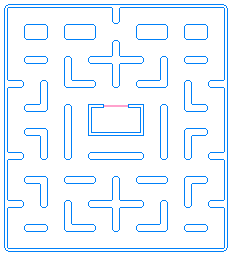保存下来，放到我们项目中Sprites文件夹中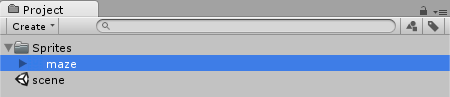然后修改它的属性。点击图片->Inspector面板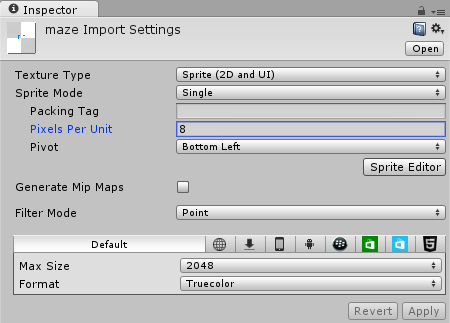注：Pixels Per Unit单位像素值设置成8，这意味着8x8像素将适合在游戏世界的一个单位。我们将使用这个值作为我们所有的纹理。我们选择了8因为两个点之间的距离总是8。我们选择左下角为枢轴因为这样以后对齐就更容易了。

### 3.吃豆人设置

• 右（边），正确的(切片0、1和2)
• 左边(第3、4及5段)
• 向上(第6、7及8段)
• 降下来(第9、10及11片)

• 右（边），正确的
• 左边
• 向上
• 降下来

``````GetComponent<Animator>().SetFloat("DirX", 0);
GetComponent<Animator>().SetFloat("DirY", 0);
``````

• 任何状态到左边有条件DirX<-0.1
• 任何状态到向上有条件DirY>0.1
• 任何状态到降下来有条件DirY<-0.1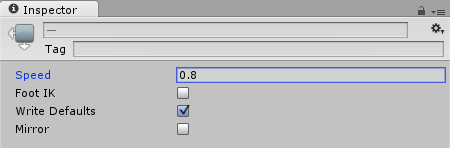添加吃豆人

``````using UnityEngine;
using System.Collections;

public class PacmanMove : MonoBehaviour {

// Use this for initialization
void Start () {

}

// Update is called once per frame
void Update () {

}
}
``````

``````using UnityEngine;
using System.Collections;

public class PacmanMove : MonoBehaviour {

void Start() {

}

void FixedUpdate() {

}
}
``````

``````using UnityEngine;
using System.Collections;

public class PacmanMove : MonoBehaviour {
public float speed = 0.4f;

void Start() {

}

void FixedUpdate() {

}
}
``````

``````bool valid(Vector2 dir) {
// Cast Line from 'next to Pac-Man' to 'Pac-Man'
Vector2 pos = transform.position;
RaycastHit2D hit = Physics2D.Linecast(pos + dir, pos);
return (hit.collider == GetComponent<Collider2D>());
}
``````

``````transform.position += dir;
``````

``````using UnityEngine;
using System.Collections;

public class PacmanMove : MonoBehaviour {
public float speed = 0.4f;
Vector2 dest = Vector2.zero;

void Start() {
dest = transform.position;
}

void FixedUpdate() {
// Move closer to Destination
Vector2 p = Vector2.MoveTowards(transform.position, dest, speed);
GetComponent<Rigidbody2D>().MovePosition(p);
}

bool valid(Vector2 dir) {
// Cast Line from 'next to Pac-Man' to 'Pac-Man'
Vector2 pos = transform.position;
RaycastHit2D hit = Physics2D.Linecast(pos + dir, pos);
return (hit.collider == GetComponent<Collider2D>());
}
}
``````

``````void FixedUpdate() {
// Move closer to Destination
Vector2 p = Vector2.MoveTowards(transform.position, dest, speed);
GetComponent<Rigidbody2D>().MovePosition(p);

// Check for Input if not moving
if ((Vector2)transform.position == dest) {
if (Input.GetKey(KeyCode.UpArrow) && valid(Vector2.up))
dest = (Vector2)transform.position + Vector2.up;
if (Input.GetKey(KeyCode.RightArrow) && valid(Vector2.right))
dest = (Vector2)transform.position + Vector2.right;
if (Input.GetKey(KeyCode.DownArrow) && valid(-Vector2.up))
dest = (Vector2)transform.position - Vector2.up;
if (Input.GetKey(KeyCode.LeftArrow) && valid(-Vector2.right))
dest = (Vector2)transform.position - Vector2.right;
}
}
``````

``````void FixedUpdate() {
// Move closer to Destination
Vector2 p = Vector2.MoveTowards(transform.position, dest, speed);
GetComponent<Rigidbody2D>().MovePosition(p);

// Check for Input if not moving
if ((Vector2)transform.position == dest) {
if (Input.GetKey(KeyCode.UpArrow) && valid(Vector2.up))
dest = (Vector2)transform.position + Vector2.up;
if (Input.GetKey(KeyCode.RightArrow) && valid(Vector2.right))
dest = (Vector2)transform.position + Vector2.right;
if (Input.GetKey(KeyCode.DownArrow) && valid(-Vector2.up))
dest = (Vector2)transform.position - Vector2.up;
if (Input.GetKey(KeyCode.LeftArrow) && valid(-Vector2.right))
dest = (Vector2)transform.position - Vector2.right;
}

// Animation Parameters
Vector2 dir = dest - (Vector2)transform.position;
GetComponent<Animator>().SetFloat("DirX", dir.x);
GetComponent<Animator>().SetFloat("DirY", dir.y);
}
``````

### 4.小圆点设置

Pacdot.cs

``````using UnityEngine;
using System.Collections;

public class Pacdot : MonoBehaviour {

// Use this for initialization
void Start () {

}

// Update is called once per frame
void Update () {

}
}
``````

``````using UnityEngine;
using System.Collections;

public class Pacdot : MonoBehaviour {

void OnTriggerEnter2D(Collider2D co) {
// Do Stuff...
}
}
``````

``````using UnityEngine;
using System.Collections;

public class Pacdot : MonoBehaviour {

void OnTriggerEnter2D(Collider2D co) {
if (co.name == "pacman")
Destroy(gameObject);
}
}
``````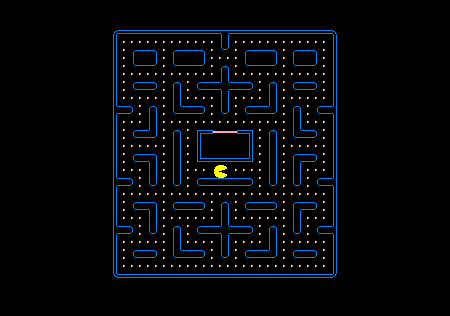### 5.敌人设置

• 右（边），正确的
• 左边
• 向上
• 降下来

``````using UnityEngine;
using System.Collections;

public class GhostMove : MonoBehaviour {

// Use this for initialization
void Start () {

}

// Update is called once per frame
void Update () {

}
}
``````

``````using UnityEngine;
using System.Collections;

public class GhostMove : MonoBehaviour {

void FixedUpdate () {

}
}
``````

``````using UnityEngine;
using System.Collections;

public class GhostMove : MonoBehaviour {
public Transform[] waypoints;

void FixedUpdate () {

}
}
``````

``````using UnityEngine;
using System.Collections;

public class GhostMove : MonoBehaviour {
public Transform[] waypoints;
int cur = 0;

void FixedUpdate () {

}
}
``````

``````using UnityEngine;
using System.Collections;

public class GhostMove : MonoBehaviour {
public Transform[] waypoints;
int cur = 0;

public float speed = 0.3f;

void FixedUpdate () {

}
}
``````

``````void FixedUpdate () {
// Waypoint not reached yet? then move closer
if (transform.position != waypoints[cur].position) {
Vector2 p = Vector2.MoveTowards(transform.position,
waypoints[cur].position,
speed);
GetComponent<Rigidbody2D>().MovePosition(p);
}
// Waypoint reached, select next one
else cur = (cur + 1) % waypoints.Length;
}
``````

``````void FixedUpdate () {
// Waypoint not reached yet? then move closer
if (transform.position != waypoints[cur].position) {
Vector2 p = Vector2.MoveTowards(transform.position,
waypoints[cur].position,
speed);
GetComponent<Rigidbody2D>().MovePosition(p);
}
// Waypoint reached, select next one
else cur = (cur + 1) % waypoints.Length;

// Animation
Vector2 dir = waypoints[cur].position - transform.position;
GetComponent<Animator>().SetFloat("DirX", dir.x);
GetComponent<Animator>().SetFloat("DirY", dir.y);
}
``````

``````void OnTriggerEnter2D(Collider2D co) {
if (co.name == "pacman")
Destroy(co.gameObject);
}
``````

• 音乐
• 分数
• 高级AI
• 开始界面
• 升级
• 生命值
• 道具奖励
• 更多动画